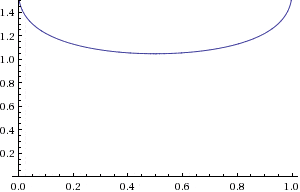# Trigonometric Equations

Simon Bridge
Homework Helper
Yep - that identity is true for all x, so if you subtract the relation you are investigating you get ... arcsin(x)+arcsin(1-x)=0 ... but I'm working on something involving the 1-2-root-3 triangle.
UPDATE: I think x could be equal to 1/2. What do you think?
It has to be - see bottom of post #24 ;) your post crossed by edit.

BUT ... plug it in: I shouldn't do this at 3am :(
arccos(0.5)=pi/3
arcsin(0.5)=pi/6
pi/3 + pi/6 = pi/2 ... but that is not the relation: pi/3-pi/6=pi/6

if arcos(x) and arcsin(1-x) are complimentary angles.
then x and 1-x correspond to the sides of a right-angle triangle.

set θ=arccos(x) and 90-θ = arcsin(1-x) are the complimentary angles right.

so x=cosθ and 1-x=sin(90-θ)=cosθ

so 1-x = x => x=1/2

The reason this got convoluted is because of the policy of helping the OP with what they are doing rather than doing the problem for them.
That was the first thing I thought of.

 don't get excited - I said if ... to use the above for your problem, you need a slight modification.
It helps understand the work so far ... see next:

Last edited:
Simon Bridge
Homework Helper
I have a feeling that either (1) the problem is misstated in post #1 OR (2) the teacher has made a mistake.

If I plot the relation to be proved, there is no solution - the there is no value of x that makes the LHS the same as the RHS.

Watch:

procede as last post:
λ=arccos(x) and θ=arcsin(1-x) so that λ - θ = 90 (working in degrees).

We notice that λ = 90+θ

from the definitions of the inverse trig functions we can write:

1-x = sinθ ...(1) and
x = cosλ = cos(90+θ) = -sinθ ... (2)

(1)+(2) gives: 1-x+x = 0 => 1=0 <=> nonsense!

Hence there is no solution.

----------------------------

LATER: I took the trouble to plot the function in gnu-octave:
Code:
octave:189> x=0:0.0125:1;
octave:190> y=acos(x)-asin(1-x);
octave:191> plot(x,y*(180/pi))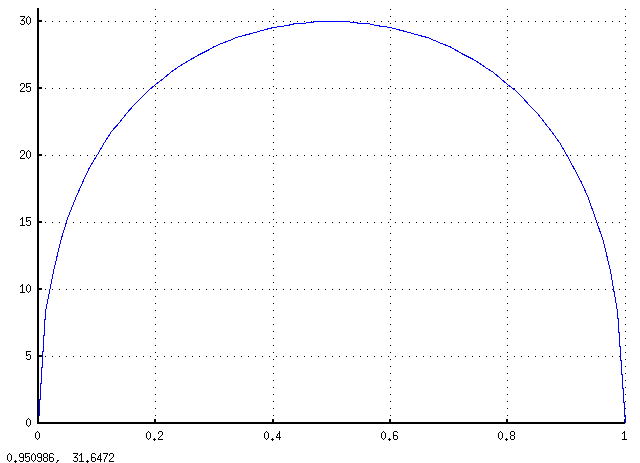... the vertical is the resultant angle and the horizontal is x. Notice that at no time does the function even get close to 90 degrees.
For x<0 octave gives complex results - so, just checking that is isn't the argument that has to be 90 degrees:
Code:
octave:199> acos(-0.5)-asin(1-(-0.5))
ans =  0.52360 - 0.96242i
octave:200> arg(ans)
ans = -1.0725
octave:201> pi/2
ans =  1.5708

#### Attachments

Last edited:
I have a feeling that either (1) the problem is misstated in post #1 OR (2) the teacher has made a mistake.

If I plot the relation to be proved, there is no solution - the there is no value of x that makes the LHS the same as the RHS.

Watch:

procede as last post:
λ=arccos(x) and θ=arcsin(1-x) so that λ - θ = 90 (working in degrees).

We notice that λ = 90+θ

from the definitions of the inverse trig functions we can write:

1-x = sinθ ...(1) and
x = cosλ = cos(90+θ) = -sinθ ... (2)

(1)+(2) gives: 1-x+x = 0 => 1=0 <=> nonsense!

Hence there is no solution.

----------------------------

LATER: I took the trouble to plot the function in gnu-octave:
Code:
octave:189> x=0:0.0125:1;
octave:190> y=acos(x)-asin(1-x);
octave:191> plot(x,y*(180/pi))... the vertical is the resultant angle and the horizontal is x. Notice that at no time does the function even get close to 90 degrees.
For x<0 octave gives complex results - so, just checking that is isn't the argument that has to be 90 degrees:
Code:
octave:199> acos(-0.5)-asin(1-(-0.5))
ans =  0.52360 - 0.96242i
octave:200> arg(ans)
ans = -1.0725
octave:201> pi/2
ans =  1.5708

I HUNDRED PERCENT AGREE TO YOU!
I tried to mathematically prove that the answer is not possible (hence no solution)

$$arccos(x) - arcsin(1-x) = 90°$$

$$arccos(x) = 90° + arcsin(1-x)$$

taking cosine of both sides:
$$x = cos90° + cos(arcsin(1-x)$$
$$x = cos[arcsin(1-x)]$$

let arcsin(1-x) = θ ⇔ sinθ = 1-x

y = 1-x x= ? r = 1

$x = \sqrt{(1)^2 - (1-x)^2}$
$x =\sqrt{(1 - (1-2x+x^2)}$
$x = \sqrt{(2x-x^2)}$

taking squares of both sides:
$x^2 = 2x+x^2$

$$x^2$$ cancels out
$$0 = 2x$$ => makes total nonsense! means there is no solution.

I have a feeling that either (1) the problem is misstated in post #1 OR (2) the teacher has made a mistake.

(1) The post is not misstated.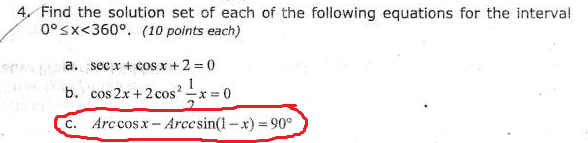(2) I feel the same. I can't confront him now because I am not scheduled today for his class. Everyone got wrong with this. So by next time we happen to see each other I'm gonna slap a paper (with this solution written) on his face!

EDIT: I probably should not do (2). I definitely don't wanna see him again next semester or take this brain-cracking course again T_T

UPDATE: Anyway sir, how do you find out the solution of trigonometric equations through graphs? Like the one you posted in post #27.

Last edited:
Mentallic
Homework Helper
$$arccos(x) = 90° + arcsin(1-x)$$

taking cosine of both sides:
$$x = cos90° + cos(arcsin(1-x)$$

Did you just do what I think you did?

To quote myself from earlier:

$$\cos(A+B)\neq \cos(A)+\cos(B)$$

Remember it's

$$\cos(A+B)=\cos(A)\cos(B)-\sin(A)\sin(B)$$

:tongue:

EDIT: By the way, even though your proof isn't correct, at the very end you had

$$0 = 2x$$ => makes total nonsense! means there is no solution.

No way! 2x=0 implies that x=0. Of course this makes sense!

Such things that would be nonsense would be if we divided by 0, or if we tried to take the square root of a negative, or the arcsin of a value outside the range -pi/2 to pi/2, or something like 1=0.

Last edited:
Did you just do what I think you did?

To quote myself from earlier:

:tongue:

Oopps. My bad. :tongue: But I will work on it again. I'm sure my answer would still be the same. I'm going to repost it to make it clear :shy:

Mentallic
Homework Helper
Oopps. My bad. :tongue: But I will work on it again. I'm sure my answer would still be the same. I'm going to repost it to make it clear :shy:

If you do it correctly, your answer should be way more nonsensical than x=0 is$$arccos(x) - arcsin(1-x) = 90°$$

$$arccos(x) = 90° + arcsin(1-x)$$

taking cosine of both sides:
$$x = cos[90° + arcsin(1-x)]$$
$$x = cos90°cos[arcsin(1-x)] - sin90°sin[arcsin(1-x)]$$
$$x = -sin[arcsin(1-x)]$$
$$x = -(1-x)$$
$$x = -1 + x$$
$$0 = -1$$
^ again, makes no sense :tongue:

Mentallic
Homework Helper
$$0 = -1$$
^ [STRIKE]again[/STRIKE], makes no sense :tongue:

Fixed. There was never a first time that you proved that it makes no sense hehe

No way! 2x=0 implies that x=0. Of course this makes sense!

Such things that would be nonsense would be if we divided by 0, or if we tried to take the square root of a negative, or the arcsin of a value outside the range -pi/2 to pi/2, or something like 1=0.

Yeah and I think I should work on remembering things :uhh:
There really is a sense in 2x = 0 (how could I say it doesn't :shy:)

Since my proof that time is senseless (because I did the wrong way of taking cosine of both sides), this x = 0 doesn't happen to be a solution right? Because what I was trying to prove is that solution is nowhere to find.

Fixed. There was never a first time that you proved that it makes no sense hehe

I was about to say that I'm referring to this:

I'm sure my answer would still be the same.

**Just wants escape the truth**

:rofl:

Mentallic
Homework Helper
Since my proof that time is senseless (because I did the wrong way of taking cosine of both sides), this x = 0 doesn't happen to be a solution right? Because what I was trying to prove is that solution is nowhere to find.

Say I want to find the solution to x such that it satisfies x+2=0, so I go about it by dividing through by 2 (incorrectly) and get x+1=0, so x=-1. Is x=-1 a solution to x+2=0?

Haha just don't let me catch you taking the cosine of a sum in the wrong way again :tongue:

Say I want to find the solution to x such that it satisfies x+2=0, so I go about it by dividing through by 2 (incorrectly) and get x+1=0, so x=-1. Is x=-1 a solution to x+2=0?

Now that's clear. I second thought because my answer in the wrong proof and right proof says the same. Now I had this conclusion that doing cosine incorrectly sometimes gives some right answers :rofl:

Haha just don't let me catch you taking the cosine of a sum in the wrong way again :tongue:

Aye aye captain! I just loved algebra that much than trigonometry. So I tend to use it on trigonometry. LOL

To forum moderators/admins, you may lock this thread now to avoid spamming. I believe it already served its purpose. Just hoping that it wont get immediately deleted due to some reason. If this thread in any way violated the rules, please inform before deleting so I can work on it. Some may find this thread useful to answer their questions. Thank you.

Also, many thanks to mentors Simon Bridge, micromass, Mentallic and Mark44 for contributing answers to this question.

Simon Bridge
Homework Helper
(1) The post is not misstated.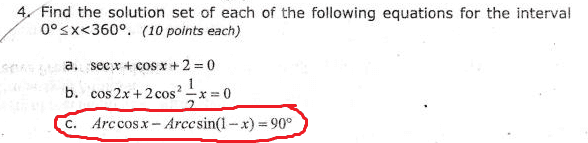(c) is the odd one out isnt it?

the given range for x is fine for the 1st two but not for (c)
"arcsin" is misspelled as arccsin.
what other carelessness is there?

UPDATE: Anyway sir, how do you find out the solution of trigonometric equations through graphs? Like the one you posted in post #27.
I used gnu-octave to plot the graph ... the solution would have been the intersection of the curve with the line y=90.

The code was:

> x=0:0.0125:1;
... says to make a vector whose first entry is 0, incrementing by 1/80 until it reaches 1;

> y=acos(x)-asin(1-x);
... this is the function I want to plot a graph of: y will be in radiens;
... the result of y will be a vector the same size as x, each entry being the result of plugging the corresponding x entry into the equation.

> plot(x,y*(180/pi))
... this plots the graph - I scales the y axis to give degrees.
... plot(X,Y) treats the two vectors X and Y as ordered pairs (Xi, Yi).

I could have plotted a line at y=90 by doing:
> plot([0,1],[90,90])
... because the plot function automatically draws a line between data points.
... plot will interpret the two vectors as the points (0,90) and (1,90).

generally: if I want to solve f(x)=c, then I could plot y=f(x) and y=c, plot both on the same axis and note the intersection ... reading x off the horizontal axis.

That is usually good for simple relations or for an approximation. For more precision, I'd use Newton-Raphson on f(x)-c=0 - using the graph to select initial values of x.

Some ability to plot the relations you are trying to solve is invaluable: it tells you what kind of solution you are aiming for. Octave is free/libre as well as free/gratis, others use Matlab or Mathematica. Only masochists use spreadsheets for stuff like this.

I guess that is pretty much all this topic exhausted - cheers.

Last edited:
(1) The post is not misstated.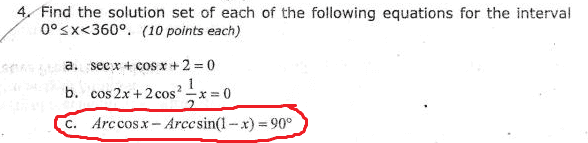(c) is the odd one out isnt it?

the given range for x is fine for the 1st two but not for (c)
"arcsin" is misspelled as arccsin.
what other carelessness is there?

I truly agree with you sir. That's why I rather choose to drop all my subjects and just study by myself here than to listen to him who even doesn't know what he's saying. He's making us fail in class by teaching us easy examples and giving out hard tests. Glad that I found this site where I can learn genuinely. The questions I am posting here in physics forum are all the hard questions which are not taught to us how to solve, yet still included in exams (yes, they're that harsh).

Anyway, GNU octave sounds very cool! Someday I would like to know how to use itEDIT: Sad to say our school might not teach us that. We're late when it comes to technology *sigh*

Last edited:
SammyS
Staff Emeritus
Homework Helper
Gold Member
https://www.physicsforums.com/attachment.php?attachmentid=50336&stc=1&d=1346433295

Well, it's easy to see how the erroneous restriction of 0° ≤ x ≤ 360° got into the problem.

That restriction makes sense for (a) & (b), but not for (c), and part (c) is what this thread is about.

The domains of the arccosine and acrsine function restrict the domain of arccos(x) - arcsin(1-x) to 0 ≤ x ≤ 1 .

Using the identity
$90^{\circ}-\arccos(x)=\arcsin(x)$​
shows that the given equation is equivalent to
$\arcsin(1-x)+\arcsin(x)=0\ .$​

A graph of y = arcsin(1-x) + arcsin(x) shows that it has no zeros. (It has no x-intercept.) So the problem has no solution, as others have pointed out.

A graph of this function from WolframAlpha may be helpful.